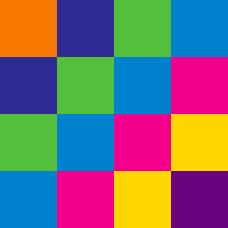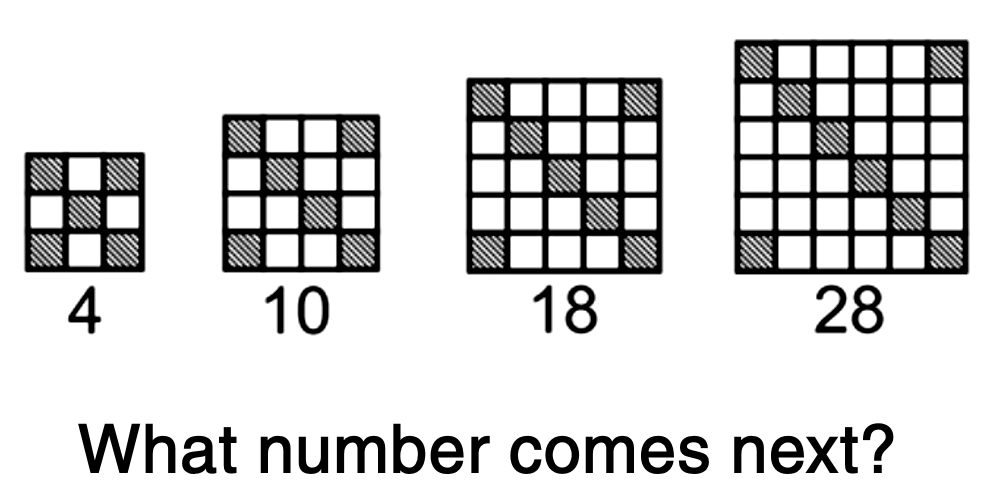Everyday Math

# Pattern Recognition

## Intro

People are natural pattern-seekers and these pattern-hunting puzzles will challenge you to think about even simple patterns in new ways!

Expect to see and learn how to solve questions like this one:In the problem above, there are many ways to notice and describe the increasing count of white squares. In particular, about 50% of people immediately recognize the pattern as an evolving or growing square and they describe it in terms of the transition from figure to figure. For example, they might solve the problem using the following logic:

“Each figure is a square, and the side length grows 1 unit every step. Since the last square shown is 6x6 units, that means the next square will be 7x7 units, or 49 units total. However, each step, the number of gray unit squares increases by 1. Therefore, since there are 8 gray squares in the last shown image, there will be 9 gray squares in the next image, so we know that the next image will have, $49 - 9 = 40$ white squares.”

This kind of description, where each figure is explained in terms of those preceding it, is known as a recursive description. However, to others, it’s more natural to think of each step as being constructed explicitly, given its position in the sequence. Given this alternate perspective, someone might solve the problem above using the following logic:

“I know I need to find the image in the 5th position this sequence. In the 1st image, the square has side-length 3, in the 2nd image, it has side length 4, etc. In general, the square in position $X$ will have side length $X+2$. Therefore, the 5th image will be a 7x7 square, composed of 49 little unit-squares. Similarly, notice that the figure in the $X^{th}$ position will always have four gray squares in the four corners and then X additional gray squares connecting the top left and lower right corners. Therefore, in the 5th image, there will be $4 + 5 = 9$ gray squares. Since $49 - 9 = 40,$ there will therefore be 40 white squares in the 5th figure."

These two types descriptions can both easily describe the given sequence and each can be the ‘more useful’ perspective to apply in different scenarios. Therefore, one can master arithmetic pattern-hunting only by both learning how to quickly identify patterns and by practicing describing these patterns in different ways and translating one type of description into another.

×